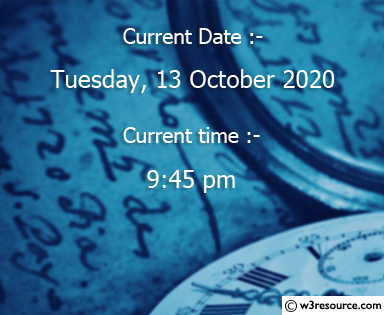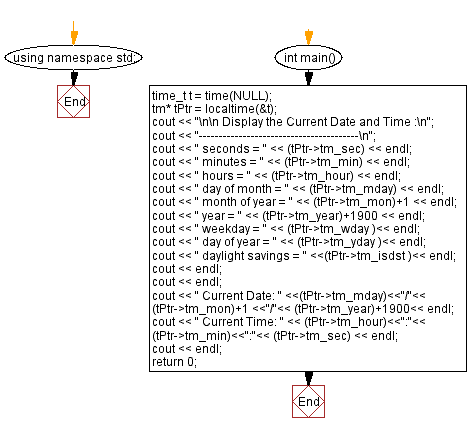﻿ C++ Exercises: Display the current date and time - w3resource

# C++ Exercises: Display the current date and time

## C++ Basic: Exercise-34 with Solution

Write a C++ program to display the current date and time.

Pictorial Presentation:Sample Solution:

C++ Code :

``````#include<iostream>
#include<cmath>
#include <ctime>
using namespace std;

int main()
{

time_t t = time(NULL);
tm* tPtr = localtime(&t);
cout << "\n\n Display the Current Date and Time :\n";
cout << "----------------------------------------\n";
cout << " seconds = " << (tPtr->tm_sec) << endl;
cout << " minutes = " << (tPtr->tm_min) << endl;
cout << " hours = " << (tPtr->tm_hour) << endl;
cout << " day of month = " << (tPtr->tm_mday) << endl;
cout << " month of year = " << (tPtr->tm_mon)+1 << endl;
cout << " year = " << (tPtr->tm_year)+1900 << endl;
cout << " weekday = " << (tPtr->tm_wday )<< endl;
cout << " day of year = " << (tPtr->tm_yday )<< endl;
cout << " daylight savings = " <<(tPtr->tm_isdst )<< endl;
cout << endl;
cout << endl;

cout << " Current Date: " <<(tPtr->tm_mday)<<"/"<< (tPtr->tm_mon)+1 <<"/"<< (tPtr->tm_year)+1900<< endl;
cout << " Current Time: " << (tPtr->tm_hour)<<":"<< (tPtr->tm_min)<<":"<< (tPtr->tm_sec) << endl;
cout << endl;
return 0;
}
``````

Sample Output:

``` Display the Current Date and Time :
----------------------------------------
seconds = 57
minutes = 33
hours = 12
day of month = 6
month of year = 7
year = 2017
weekday = 4
day of year = 186
daylight savings = 0

Current Date: 6/7/2017
Current Time: 12:33:57
```

Flowchart:C++ Code Editor:

What is the difficulty level of this exercise?

﻿

## C++ Programming: Tips of the Day

Why is there no std::stou?

The most pat answer would be that the C library has no corresponding "strtou", and the C++11 string functions are all just thinly veiled wrappers around the C library functions: The std::sto* functions mirror strto*, and the std::to_string functions use sprintf.

Ref: https://bit.ly/3wtz2qA

We are closing our Disqus commenting system for some maintenanace issues. You may write to us at reach[at]yahoo[dot]com or visit us at Facebook